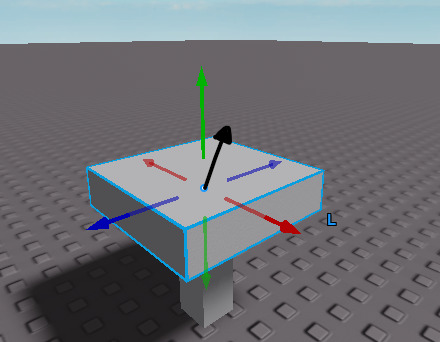# Rotate Weld.C0 on Specific Axis

I am looking to rotate the C0 of a weld on a specific axis. Not along the Global or Local axis but on an undetermined axis.

An example being if I wanted to rotate this part in the direction the black arrow is facing
So that it would rotate from this:To this:I am having trouble figuring out the CFrame math for this. Any help would be appreciated!

This should work, derived it from formula in my CFrame tutorial.

The rotation should occur in any axis however it’s set to the center of part1 to a target point in this case, and the rotation is set at the center of the part1, let me know if you want to change the center of rotation.

It uses world space axis rotation howeverr with a specified axis using CFrame.fromAxisAngles:

``````--Evaera arrow adornment
--https://gist.github.com/evaera/735f23c67c2c173cfed2955f357b03a3
local function arrow(name, from, to, color, scale, alwaysOnTop : boolean)
if alwaysOnTop == nil then
alwaysOnTop = true
end

color = color or BrickColor.random().Color
scale = scale or 1

if typeof(from) == "Instance" then
if from:IsA("BasePart") then

from = from.CFrame
elseif from:IsA("Attachment") then
from = from.WorldCFrame
end

if to ~= nil then
from = from.p
end
end

if typeof(to) == "Instance" then
if to:IsA("BasePart") then
to = to.Position
elseif to:IsA("Attachment") then
to = to.WorldPosition
end
end

if typeof(from) == "CFrame" and to == nil then
local look = from.lookVector
to = from.p
from = to + (look * -10)
end

if to == nil then
to = from
from = to + Vector3.new(0, 10, 0)
end

assert(typeof(from) == "Vector3" and typeof(to) == "Vector3", "Passed parameters are of invalid types")

local container = workspace:FindFirstChild("Arrows") or Instance.new("Folder")
container.Name = "Arrows"
container.Parent = workspace

local shaft = container:FindFirstChild(name .. "_shaft") or Instance.new("CylinderHandleAdornment")

shaft.Height = (from - to).magnitude - 2

shaft.CFrame = CFrame.lookAt(
((from + to)/2) - ((to - from).unit * 1),
to
)

if shaft.Parent == nil then
shaft.Name = name .. "_shaft"
shaft.Color3 = color
shaft.Transparency = 0
shaft.Transparency = 0
shaft.AlwaysOnTop = alwaysOnTop
shaft.ZIndex = 5 - math.ceil(scale)
end

shaft.Parent = container

scale = scale == 1 and 1 or 1.4

if pointy.Parent == nil then
pointy.Color3 = color
pointy.Transparency = 0
pointy.Height = 2 * scale
pointy.AlwaysOnTop = alwaysOnTop
pointy.ZIndex = 5 - math.ceil(scale)
end

pointy.CFrame = CFrame.lookAt((CFrame.lookAt(to, from) * CFrame.new(0, 0, -2 - ((scale-1)/2))).p, to)

pointy.Parent = container

if scale == 1 then
arrow(name .. "_backdrop", from, to, Color3.new(0, 0, 0), 2, alwaysOnTop)
end

return shaft, pointy
end

local weld = script.Parent
local part1 = weld.Part1
local targetPoint = workspace.Target

local function worldCFrameRotationToC0ObjectSpace(motor6DJoint,worldCFrame)
local part0 = motor6DJoint.Part0
local c1Store = motor6DJoint.C1
local c0Store = motor6DJoint.C0

local relativeToPart0 = part0.CFrame:inverse() * worldCFrame * c1Store

return relativeToPart0
end

local dt = 0.0001

targetPoint.Position = part1.Position+Vector3.new(0,5,0)

while true do
local rotationAxis = (targetPoint.Position - part1.Position)
arrow("Test", part1, targetPoint.Position, nil, nil, false)
local rotationCFrame = CFrame.fromAxisAngle(rotationAxis.Unit,dt*2.5)

local goalWorldSpaceCFrame = (rotationCFrame*part1.CFrame).Rotation + part1.CFrame.Position
weld.C0 = worldCFrameRotationToC0ObjectSpace(weld, goalWorldSpaceCFrame)